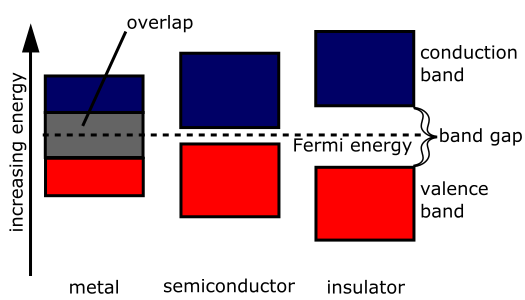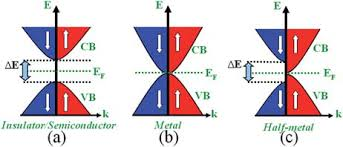求倒格矢

$$\begin{cases} b_{1}=2\pi \frac {a_{2}\times a_{3} }{ a_{1}\cdot ( a_{2}\times a_{3})}; \ b_{2}=2\pi \frac { a_{3}\times a_{1}}{ a_{2}\cdot ( a_{3}\times a_{1})};\ b_{3}=2\pi \frac { a_{1}\times a_2}{ a_{3}\cdot ( a_1\times a_2)}; \end{cases}$$

$$a_{i}\cdot b_{j}=2\pi \delta _{ij}= \begin{cases} 2\pi ,&i\ =\ j;\ 0,&i\ \neq \ j; \end{cases}$$

固体的结合

• 金属结合

LA、ILA族及过渡元素，它们的电负性小，最外层一般有一两个容易失去的价电子.构成元素晶体时，晶格上既有金属原子，又有失去了电子的金属离子.但它们都是不稳定的.价电子会向正金属离子运动，即金属离子随时会变成金属原子，金属原子随时会变成金属离子.价电子不再属于个别原子，而是为所有原子所共有，在晶体中作共有化运动.采用一个更简化的物理模型：金属中所有的原子都失掉了最外层的价电子而成为原子实，原子实浸没在共有电子的电子云中.金属晶体的结合力主要是原子实和共有化电子之间的静电库仑力.金属结合只受最小能量的限制，原子越紧凑，电子云与原子实就越紧密，库仑能就越低.

• 共价结合

• 离子结合

• 范德瓦尔斯结合

（1）极性分子间的结合

（2）极性分子与非极性分子的结合

（3）非极性分子间的结合

• 氢键结合

能带论• 金属的能带穿过费米能级
• 半导体的能到不穿过，且禁带宽度较小
• 绝缘体的能带也不穿过费米能级，禁带宽度大
• 半金属（semi-mental)的能带与费米能级相切。
• 半金属（half-mental)自旋向上部分与金属相同，自旋向下部分与绝缘体相同。

Kohn-Sham 方程

$$F=F(n)$$

• 费米子构成物质
• 玻色子传递相互作用

Slater 行列式(N=2)。

2010 年物理学诺贝尔奖与石墨烯Donate comment here